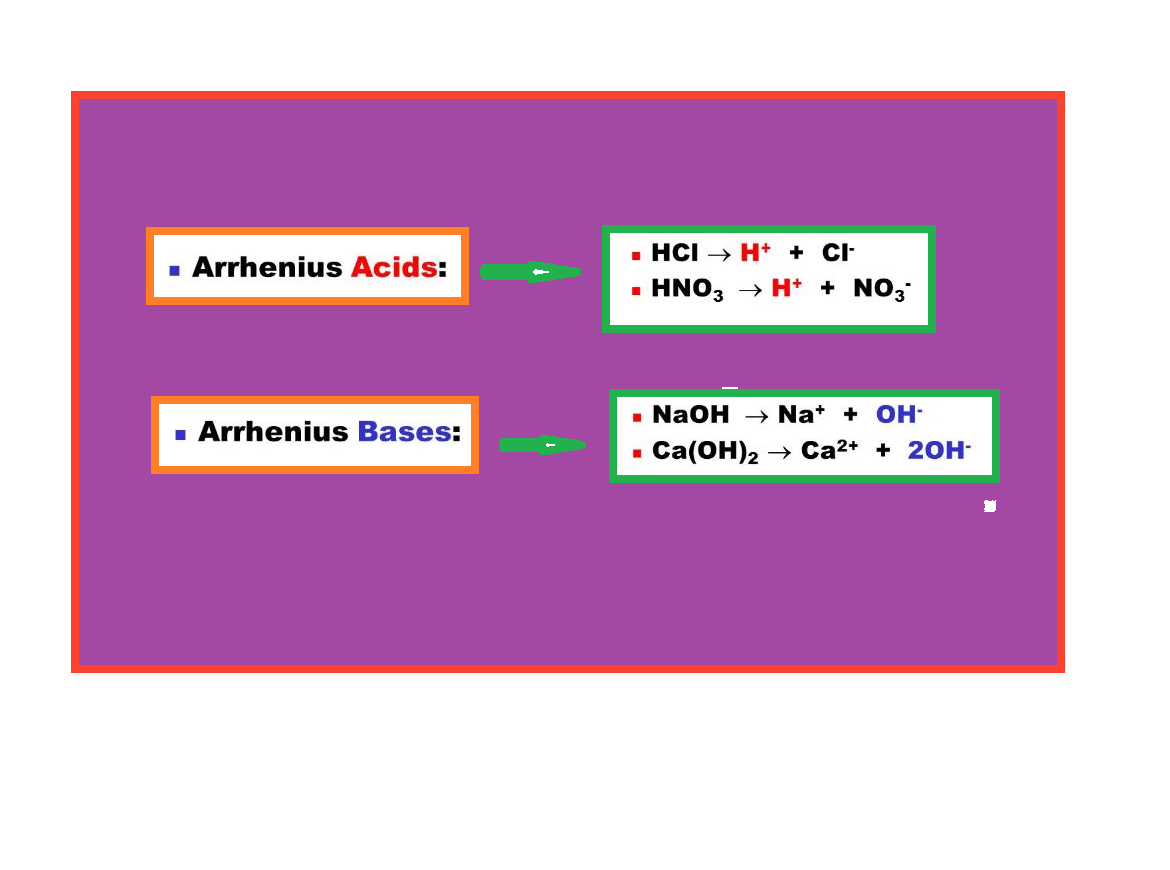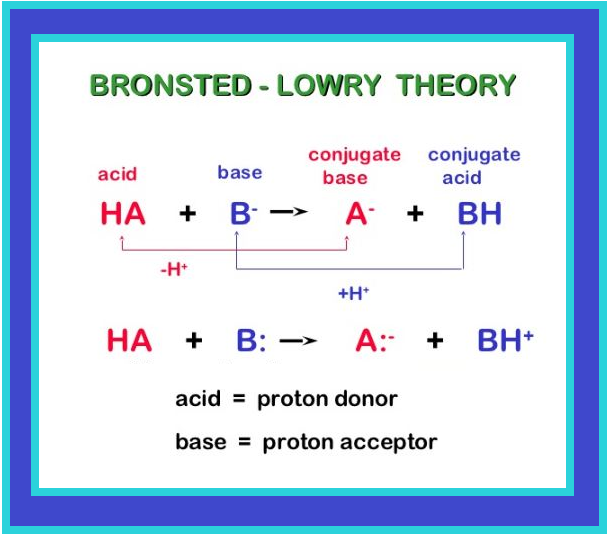Chemistry IONIC EQUILIBRIUM, ACID AND BASE THEORY

### Topic to be covered

star IONIC EQUILIBRIUM IN SOLUTION .
star ACIDS, BASES AND SALTS.
star The Brönsted-Lowry Acids and Bases.
star Arrhenius Concept of Acids and Bases.
star Lewis Acids and Bases

### IONIC EQUILIBRIUM IN SOLUTION=> Under the effect of change of concentration on the direction of equilibrium, you have incidently come across with the following equilibrium which involves ions:

color{red}(Fe^(3+) (aq) +SCN^(-) (aq) ⇌ [Fe(SCN)]^(2+) (aq))

There are numerous equilibria that involve ions only.

=> It is well known that the aqueous solution of sugar does not conduct electricity. However, when common salt (sodium chloride) is added to water it conducts electricity. Also, the conductance of electricity increases with an increase in concentration of common salt.

=> Michael Faraday classified the substances into two categories based on their ability to conduct electricity.
•One category of substances conduct electricity in their aqueous solutions and are called electrolytes.
•while the other do not and are thus, referred to as nonelectrolytes.

=> Faraday further classified electrolytes into strong and weak electrolytes.
• Strong electrolytes on dissolution in water are ionized almost completely.
• The weak electrolytes are only partially dissociated.

For example, an aqueous solution of sodium chloride is comprised entirely of sodium ions and chloride ions(there is almost 100% ionization in case of sodium chloride ), while that of acetic acid mainly contains unionized acetic acid molecules and only some acetate ions and hydronium ions( less than 5% ionization of acetic acid which is a weak electrolyte). It should be noted that in weak electrolytes, equilibrium is established between ions and the unionized molecules.

This type of equilibrium involving ions in aqueous solution is called ionic equilibrium. Acids, bases and salts come under the category of electrolytes and may act as either strong or weak electrolytes.

### ACIDS, BASES AND SALTS• Hydrochloric acid present in the gastric juice is secreted by the lining of our stomach in a significant amount of 1.2-1.5 L/day and is essential for digestive processes.
• Acetic acid is known to be the main constituent of vinegar.
•Lemon and orange juices contain citric and ascorbic acids.
•Tartaric acid is found in tamarind paste.

color{green}("PROPERTIES OF ACIDS")

=> As most of the acids taste sour, the word “acid” has been derived from a latin word “acidus” meaning sour.
=> Acids are known to turn blue litmus paper into red and liberate dihydrogen on reacting with some metals.

color{green}("PROPERTIES OF BASES")

=> Bases are known to turn red litmus paper blue
=> They taste bitter and
=> They feel soapy.
=> A common example of a base is washing soda used for washing purposes.

• When acids and bases are mixed in the right proportion they react with each other to give salts. Some commonly known examples of salts are sodium chloride, barium sulphate, sodium nitrate. Sodium chloride (common salt ) is an important component of our diet and is formed by reaction between hydrochloric acid and sodium hydroxide. It exists in solid state as a cluster of positively charged sodium ions and negatively charged chloride ions which are held together due to electrostatic interactions between oppositely charged species (Fig.7.10).
•The electrostatic forces between two charges are inversely proportional to dielectric constant of the medium.
• Water, a universal solvent, possesses a very high dielectric constant of 80. Thus, when sodium chloride is dissolved in water, the electrostatic interactions are reduced by a factor of 80 and this facilitates the ions to move freely in the solution. Also, they are well separated due to hydration with water molecules.

The extent to which ionization occurs depends upon the strength of the bond and the extent of solvation of ions produced. The terms dissociation and ionization have earlier been used with different meaning.

color { maroon} ® color{maroon} ul (" REMEMBER")

Dissociation refers to the process of separation of ions in water already existing as such in the solid state of the solute, as in sodium chloride. On the other hand, ionization corresponds to a process in which a neutral molecule splits into charged ions in the solution. Here, we shall not distinguish between the two and use the two terms interchangeably.### Arrhenius Concept of Acids and Basescolor{purple}(✓✓)color{purple} " DEFINITION ALERT"

According to Arrhenius theory, acids are substances that dissociates in water to give hydrogen ions color{red}(H^+ (aq)) and bases are substances that produce hydroxyl ions color{red}(OH^–(aq)). The ionization of an acid color{red}(HX (aq)) can be represented by the following equations:

color{red}(HX (aq) → H^+ (aq) +X^(-) (aq))

or color{red}(HX (aq) +H_2O(l) → H_3O^(+) (aq) + X^(-) (aq))

A bare proton, color{red}(H^+) is very reactive and cannot exist freely in aqueous solutions. Thus, it bonds to the oxygen atom of a solvent water molecule to give trigonal pyramidal hydronium ion, color{red}(H_3O^+ {[H (H_2O)]^+}) (see box). In this chapter we shall use color{red}(H^+(aq)) and color{red}(H_3O^+(aq)) interchangeably to mean the same i.e., a hydrated proton.

Similarly, a base molecule like color{red}(MOH) ionizes in aqueous solution according to the equation:

color{red}(MOH (aq) → M^+(aq) +OH^(-) (aq))

The hydroxyl ion also exists in the hydrated form in the aqueous solution. Arrhenius concept of acid and base, however, suffers from the limitation of being applicable only to aqueous solutions and also, does not account for the basicity of substances like, ammonia which do not possess a hydroxyl group.### The Brönsted-Lowry Acids and Basescolor{purple}(✓✓)color{purple} " DEFINITION ALERT"

According to Brönsted-Lowry theory, acid is a substance that is capable of donating a hydrogen ion color{red}(H^+) and bases are substances capable of accepting a hydrogen ion, color{red}(H^+). In short, acids are proton donors and bases are proton acceptors.

Consider the example of dissolution of color{red}(NH_3) in color{red}(H_2O) represented by the following equation:

color{red}(undersettext(base)(NH_3(aq)) +undersettext(acid)(H_2O(I)) ⇌ undersettext(conjugate acid)(NH_4^(+)(aq)) + undersettext(conjugate base)(OH^(-) (aq)))

color{red}(NH_3(aq) oversettext(adds proton)→ undersettext(conjugate acid)(NH_4^(+)(aq)))

color{red}(undersettext(acid)(H_2O(l)) oversettext(loses proton) → undersettext(conjugate base)(OH^(-)(aq)))

•The basic solution is formed due to the presence of hydroxyl ions. In this reaction, water molecule acts as proton donor and ammonia molecule acts as proton acceptor and are thus, called Lowry-Brönsted acid and base, respectively.

•In the reverse reaction, color{red}(H^+) is transferred from color{red}(NH_4^(+)) to color{red}(OH^–). In this case, color{red}(NH_4^+) acts as a Bronsted acid while color{red}(OH^–) acted as a Brönsted base.

•The acid-base pair that differs only by one proton is called a conjugate acid-base pair. Therefore, color{red}(OH^–) is called the conjugate base of an acid color{red}(H_2O) and color{red}(NH_4^+) is called conjugate acid of the base color{red}(NH_3).

•If Brönsted acid is a strong acid then its conjugate base is a weak base and viceversa. It may be noted that conjugate acid has one extra proton and each conjugate base has one less proton.

Consider the example of ionization of hydrochloric acid in water. color{red}(HCl(aq)) acts as an acid by donating a proton to color(H_2O) molecule which acts as a base.

color{red}(undersettext(acid)(HCl(aq)) + undersettext(base)(H_2O(l)) ⇌ undersettext(conjugate acid)(H_3O^(+)(aq)) + undersettext(conjugate base)(Cl^(-) (aq)))

color{red}(undersettext(acid)(HCl(aq)) oversettext(loses proton)→ undersettext(conjugate base)(Cl^(-)(aq)))

color{red}(undersettext(base)(H_2O(l)) oversettext(adds proton) → undersettext(conjugate acid)(H_3O^(+)(aq)))

It can be seen in the above equation, that water acts as a base because it accepts the proton. The species color{red}(H_3O^+) is produced when water accepts a proton from color{red}(HCl). Therefore, color{red}(Cl^–) is a conjugate base of color{red}(HCl) and color{red}(HCl) is the conjugate acid of base color{red}(Cl^–). Similarly, color{red}(H_2O) is a conjugate base of an acid color{red}(H_3O^+) and color{red}(H_3O^+) is a conjugate acid of base color{red}(H_2O).

It is interesting to observe the dual role of water as an acid and a base. In case of reaction with color{red}(HCl) water acts as a base while in case of ammonia it acts as an acid by donating a proton.Q 3019891719What will be the conjugate bases for the following Brönsted acids: HF, H_2SO_4 and HCO_3^– ?Solution:

The conjugate bases should have one proton less in each case and therefore the corresponding conjugate bases are: F^–,HSO_4^-
and CO_3^(2–) respectively.
Q 3059091814Write the conjugate acids for the following Brönsted bases: NH_2^–, NH_3 and HCOO^–.Solution:

The conjugate acid should have one extra proton in each case and therefore the corresponding conjugate acids are: NH_3,
NH_4^+ and HCOOH respectively.
Q 3079191916The species: H_2O, HCO_3^–, HSO_4^– and NH_3 can act both as Bronsted acids and bases. For each case give the corresponding
conjugate acid and conjugate base.Solution:

The answer is given in the following Table:

tt(( text{Species} , text{Conjugate acid} , text{Conjugate base} ) , ( H_2O , H_3O^+ , OH^- ) , (HCO_3^- , H_2CO_3 , CO_3^(2-) ) , ( HSO_4^(-) , H_2SO_4 , SO_4^(2-)), (NH_3 , NH_4^+ , NH_2))

### Lewis Acids and BasesG.N. Lewis in 1923 defined an acid as a species which accepts electron pair and base which donates an electron pair. As far as bases are concerned, there is not much difference between Brönsted-Lowry and Lewis concepts, as the base provides a lone pair in both the cases. However, in Lewis concept many acids do not have proton. A typical example is reaction of electron deficient species color{red}(BF_3) with color{red}(NH_3).

color{red}(BF_3) does not have a proton but still acts as an acid and reacts with color{red}(NH_3) by accepting its lone pair of electrons. The reaction can be represented by,

color{red}(BF_3 + : NH_3 → BF_3 : NH_3)

Electron deficient species like color{red}(AlCl_3, Co^(3+), Mg^(2+)), etc. can act as Lewis acids while species like color{red}(H_2O ,NH_3 ,OH^–) etc. which can donate a pair of electrons, can act as Lewis bases.

Q 3150101014Classify the following species into Lewis acids and Lewis bases and show how these act as such:

(a) HO^- , (b) F^- , (c) H^+ , (d) BCl_3Solution:

(a) Hydroxyl ion is a Lewis base as it can donate an electron lone pair ( : OH^–).

(b) Flouride ion acts as a Lewis base as it can donate any one of its four electron lone pairs.

(c) A proton is a Lewis acid as it can accept a lone pair of electrons from bases like hydroxyl ion and fluoride ion.

(d) BCl_3 acts as a Lewis acid as it can accept a lone pair of electrons from species like ammonia or amine molecules.